# AP Calculus AB Practice Test 51

### Test Information10 questions20 minutes

Calculator Disallowed

1.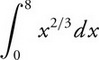2. The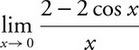is :

3. What is the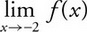, if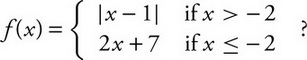4. The graph of f′ is shown below.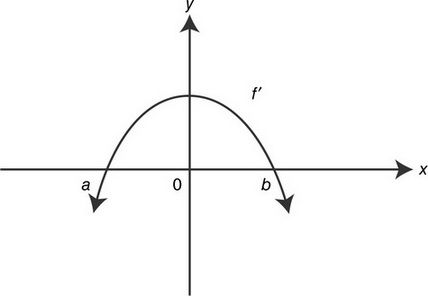Which of the graphs below is a possible graph of f?

5.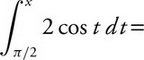6. Given the equation y= 3e-2x, what is an equation of the normal line to the graph at x= ln 2?

7. What is the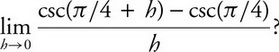8. If f(x)is an antiderivative of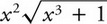and f(2) = 0, then f(0) =

9. If a function fis continuous for all values of x, which of the following statements is/are always true?

I.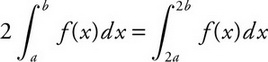II.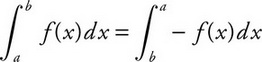III.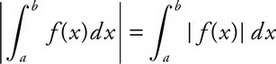10. The graph of fis shown below and fis twice differentiable. Which of the following has the largest value: f(0), f′(0), f″(0)?